Updating search results...# Grade 8 DLC Blended Math

This collection features resources developed by The Distance Learning Centre (DLC) for blended math in Grade 8.

51 affiliated resources

# Search Resources

View
Selected filters: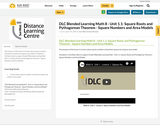Conditional Remix & Share Permitted
CC BY-NC-SA
Rating
0.0 stars

The purpose of this lesson is to learn about square numbers and perfect squares by using an area model.

Included is a YouTube video to support Grade 8 Blended Learning Math - Unit 1.1: Square Roots and Pythagorean Theorem - Square Numbers and Area Models.

Subject:
Math
Material Type:
Activity/Lab
Homework/Assignment
Lesson
Provider:
Sun West Distance Learning Centre (DLC)
12/05/2023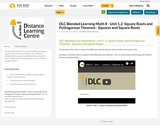Conditional Remix & Share Permitted
CC BY-NC-SA
Rating
0.0 stars

The purpose of this lesson is to learn the difference between squares, square numbers and square roots.

Included is a YouTube video to support Grade 8 Blended Learning Math - Unit 1.2: Square Roots and Pythagorean Theorem - Squares and Square Roots.

Subject:
Math
Material Type:
Activity/Lab
Homework/Assignment
Lesson
Provider:
Sun West Distance Learning Centre (DLC)
12/05/2023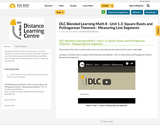Conditional Remix & Share Permitted
CC BY-NC-SA
Rating
0.0 stars

The purpose of this lesson is to learn about we can use the properties of a square to find its area or side length.

Included is a YouTube video to support Grade 8 Blended Learning Math - Unit 1.3: Square Roots and Pythagorean Theorem - Measuring Line Segments.

Subject:
Math
Material Type:
Activity/Lab
Homework/Assignment
Lesson
Provider:
Sun West Distance Learning Centre (DLC)
12/05/2023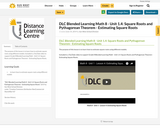Conditional Remix & Share Permitted
CC BY-NC-SA
Rating
0.0 stars

The purpose of this lesson is to learn how to estimate square roots using different models.

Included is a YouTube video to support Grade 8 Blended Learning Math - Unit 1.4: Square Roots and Pythagorean Theorem - Estimating Square Roots.

Subject:
Math
Material Type:
Activity/Lab
Homework/Assignment
Lesson
Provider:
Sun West Distance Learning Centre (DLC)
12/05/2023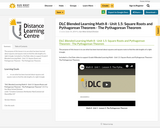Conditional Remix & Share Permitted
CC BY-NC-SA
Rating
0.0 stars

The purpose of this lesson is to use what has been learned about squares and square roots to find the side lengths of a right triangle.

Included is a YouTube video to support Grade 8 Blended Learning Math - Unit 1.5: Square Roots and Pythagorean Theorem - The Pythagorean Theorem.

Subject:
Math
Material Type:
Activity/Lab
Homework/Assignment
Lesson
Provider:
Sun West Distance Learning Centre (DLC)
12/05/2023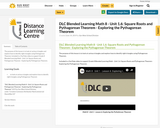Conditional Remix & Share Permitted
CC BY-NC-SA
Rating
0.0 stars

The purpose of this lesson is to look at various triangles and explore how to identify right triangles using Pythagorean Theorem.

Included is a YouTube video to support Grade 8 Blended Learning Math - Unit 1.6: Square Roots and Pythagorean Theorem - Exploring the Pythagorean Theorem.

Subject:
Math
Material Type:
Activity/Lab
Homework/Assignment
Lesson
Provider:
Sun West Distance Learning Centre (DLC)
12/05/2023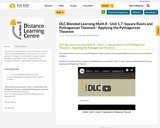Conditional Remix & Share Permitted
CC BY-NC-SA
Rating
0.0 stars

The purpose of this lesson is to use the Pythagorean Theorem and its corresponding algebraic expression solve a variety of problems.

Included is a YouTube video to support Grade 8 Blended Learning Math - Unit 1.7: Square Roots and Pythagorean Theorem - Applying the Pythagorean Theorem.

Subject:
Math
Material Type:
Activity/Lab
Homework/Assignment
Lesson
Provider:
Sun West Distance Learning Centre (DLC)
12/05/2023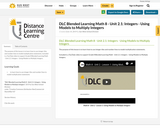Conditional Remix & Share Permitted
CC BY-NC-SA
Rating
0.0 stars

The purpose of this lesson is to learn how to use integer tiles and number lines to model multiplication statements.

Included is a YouTube video to support Grade 8 Blended Learning Math - Unit 2.1: Integers - Using Models to Multiply Integers.

Subject:
Math
Material Type:
Activity/Lab
Homework/Assignment
Lesson
Provider:
Sun West Distance Learning Centre (DLC)
12/05/2023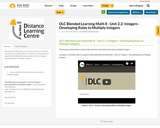Conditional Remix & Share Permitted
CC BY-NC-SA
Rating
0.0 stars

The purpose of this lesson is to learn about the four rules that can be used to multiply integers.

Included is a YouTube video to support Grade 8 Blended Learning Math - Unit 2.2: Integers - Developing Rules to Multiply Integers.

Subject:
Math
Material Type:
Activity/Lab
Homework/Assignment
Lesson
Provider:
Sun West Distance Learning Centre (DLC)
12/05/2023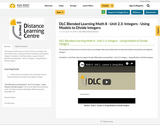Conditional Remix & Share Permitted
CC BY-NC-SA
Rating
0.0 stars

The purpose of this lesson is to learn how to use integer tiles and number lines to represent division of positive and negative integers.

Included is a YouTube video to support Grade 8 Blended Learning Math - Unit 2.3: Integers - Using Models to Divide Integers.

Subject:
Math
Material Type:
Activity/Lab
Homework/Assignment
Lesson
Provider:
Sun West Distance Learning Centre (DLC)
12/05/2023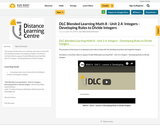Conditional Remix & Share Permitted
CC BY-NC-SA
Rating
0.0 stars

The purpose of this lesson is to develop some rules to help with the dividing of positive and negative integers.

Included is a YouTube video to support Grade 8 Blended Learning Math - Unit 2.4: Integers - Developing Rules to Divide Integers.

Subject:
Math
Material Type:
Activity/Lab
Homework/Assignment
Lesson
Provider:
Sun West Distance Learning Centre (DLC)
12/05/2023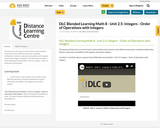Conditional Remix & Share Permitted
CC BY-NC-SA
Rating
0.0 stars

The purpose of this lesson is to learn how to solve problems that include several different operations, including multiplication, division, subtraction and addition with negative and positive integers.

Included is a YouTube video to support Grade 8 Blended Learning Math - Unit 2.5: Integers - Order of Operations with Integers.

Subject:
Math
Material Type:
Activity/Lab
Homework/Assignment
Lesson
Provider:
Sun West Distance Learning Centre (DLC)
12/05/2023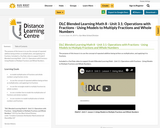Conditional Remix & Share Permitted
CC BY-NC-SA
Rating
0.0 stars

The purpose of this lesson is to use the concept of repeated addition being written as multiplication, and applying it to fractions.

Included is a YouTube video to support Grade 8 Blended Learning Math - Unit 3.1: Operations with Fractions - Using Models to Multiply Fractions and Whole Numbers.

Subject:
Math
Material Type:
Activity/Lab
Homework/Assignment
Lesson
Provider:
Sun West Distance Learning Centre (DLC)
12/05/2023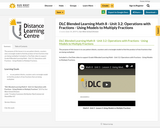Conditional Remix & Share Permitted
CC BY-NC-SA
Rating
0.0 stars

The purpose of this lesson is to use pattern blocks, counters and a rectangle model to find the product of two fractions that are being multiplied.

Included is a YouTube video to support Grade 8 Blended Learning Math - Unit 3.2: Operations with Fractions - Using Models to Multiply Fractions.

Subject:
Math
Material Type:
Activity/Lab
Homework/Assignment
Lesson
Provider:
Sun West Distance Learning Centre (DLC)
12/05/2023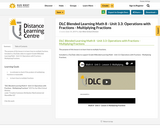Conditional Remix & Share Permitted
CC BY-NC-SA
Rating
0.0 stars

The purpose of this lesson is to learn how to multiply fractions.

Included is a YouTube video to support Grade 8 Blended Learning Math - Unit 3.3: Operations with Fractions - Multiplying Fractions.

Subject:
Math
Material Type:
Activity/Lab
Homework/Assignment
Lesson
Provider:
Sun West Distance Learning Centre (DLC)
12/05/2023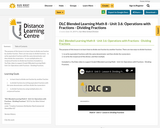Conditional Remix & Share Permitted
CC BY-NC-SA
Rating
0.0 stars

The purpose of this lesson is to learn how to divide one fraction by another fraction. There are two ways to divide fractions:
- to write equivalent fractions with the same denominator and then divide the numerators
- to use multiplication of a reciprocal fraction to divide two fractions

Included is a YouTube video to support Grade 8 Blended Learning Math - Unit 3.6: Operations with Fractions - Dividing Fractions.

Subject:
Math
Material Type:
Activity/Lab
Homework/Assignment
Lesson
Provider:
Sun West Distance Learning Centre (DLC)
12/05/2023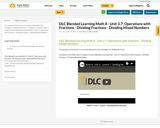Conditional Remix & Share Permitted
CC BY-NC-SA
Rating
0.0 stars

The purpose of this lesson is to review and practice the strategies for dividing fractions .

Included is a YouTube video to support Grade 8 Blended Learning Math - Unit 3.7: Operations with Fractions - Dividing Fractions - Dividing Mixed Numbers.

Subject:
Math
Material Type:
Activity/Lab
Homework/Assignment
Lesson
Provider:
Sun West Distance Learning Centre (DLC)
12/05/2023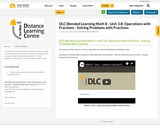Conditional Remix & Share Permitted
CC BY-NC-SA
Rating
0.0 stars

The purpose of this lesson is to use various operations to solve word problems involving fractions.

Included is a YouTube video to support Grade 8 Blended Learning Math - Unit 3.8: Operations with Fractions - Solving Problems with Fractions.

Subject:
Math
Material Type:
Activity/Lab
Homework/Assignment
Lesson
Provider:
Sun West Distance Learning Centre (DLC)
12/05/2023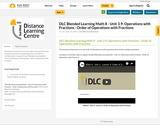Conditional Remix & Share Permitted
CC BY-NC-SA
Rating
0.0 stars

The purpose of this lesson is to use order of operations to solve questions that involve multiple operations.

Included is a YouTube video to support Grade 8 Blended Learning Math - Unit 3.9: Operations with Fractions - Order of Operations with Fractions.

Subject:
Math
Material Type:
Activity/Lab
Homework/Assignment
Lesson
Provider:
Sun West Distance Learning Centre (DLC)
12/05/2023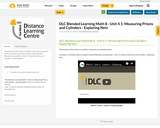Conditional Remix & Share Permitted
CC BY-NC-SA
Rating
0.0 stars

The purpose of this lesson is to explore using nets to represent prisms.

Included is a YouTube video to support Grade 8 Blended Learning Math - Unit 4.1: Measuring Prisms and Cylinders - Exploring Nets.

Subject:
Math
Material Type:
Activity/Lab
Homework/Assignment
Lesson
Provider:
Sun West Distance Learning Centre (DLC)